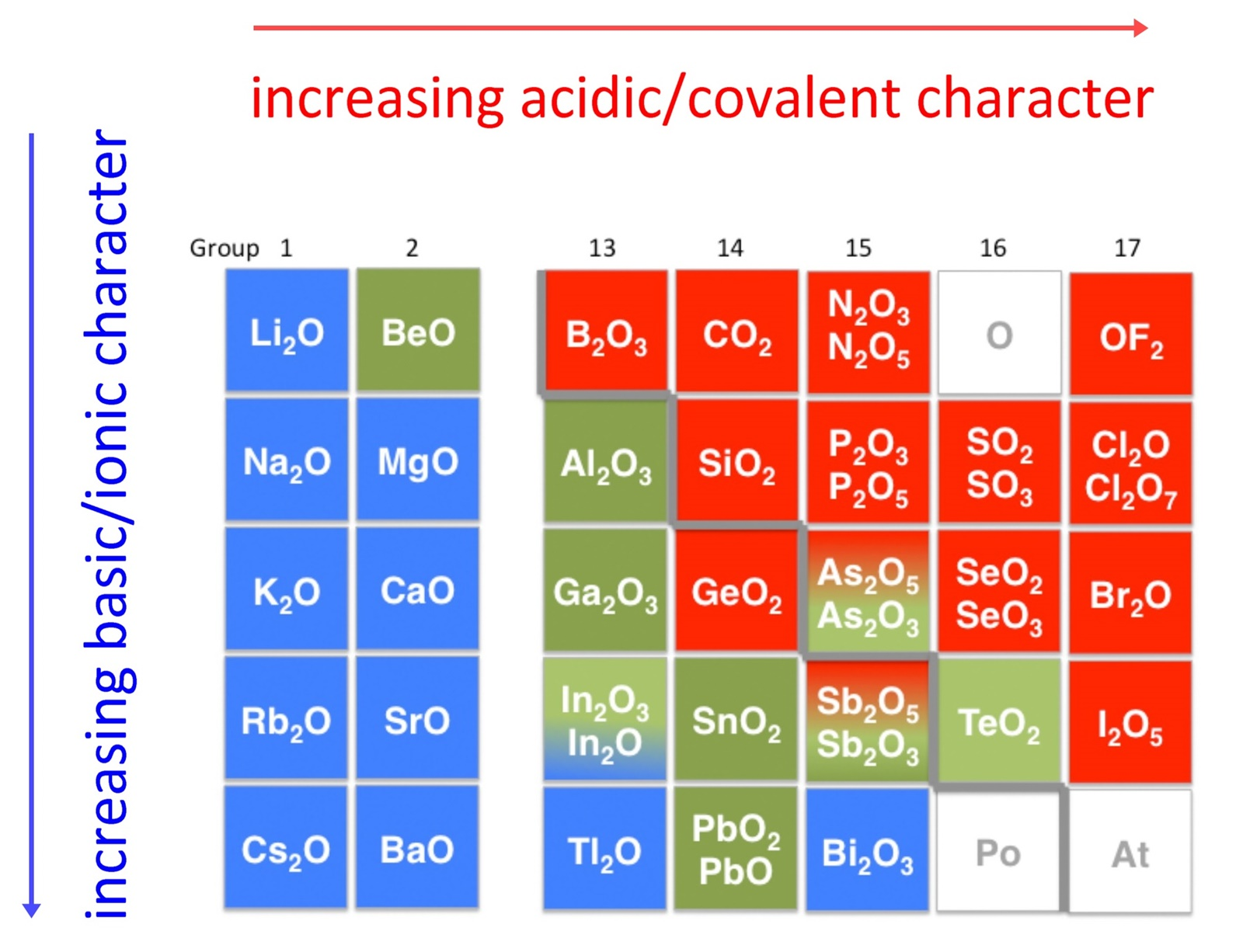7.8A: Amphoteric Behavior

Oxides and Hydroxides

An early classification of substances arose from the differences observed in their solubility in acidic and basic solutions. This led to the classification of oxides and hydroxides as being either acidic or basic. Acidic oxides or hydroxides either reacted with water to produce an acidic solution or were soluble in aqueous base. Basic oxides and hydroxides either reacted with water to produce a basic solution or readily dissolved in aqueous acids. The diagram below shows there is strong correlation between the acidic or basic character of oxides (ExOy) and the position of the element, E, in the periodic table.

Oxides of metallic elements are generally basic oxides, and oxides of nonmetallic elements acidic oxides. Take for example, the reactions with water of calcium oxide, a metallic oxide, and carbon dioxide, a nonmetallic oxide:

CaO(s) + H2O(l) → Ca(OH)2
CO2(g) + H2O(l) → H2CO3(aq)
Calcium oxide reacts with water to produce a basic solution of calcium hydroxide, whereas carbon dioxide reacts with water to produce a solution of carbonic acid.There is a gradual transition from basic oxides to acidic oxides from the lower left to the upper right in the periodic table.

Basicity of the oxides increase with increasing atomic number down a group:
BeO < MgO < CaO < SrO < BaO
Note as well that acidity increases with increasing oxidation state of the element:
MnO < Mn2O3 < MnO2 < Mn2O7
in keeping with the increase in covalency.
Oxides of intermediate character, called amphoteric oxides, are located along the diagonal line between the two extremes. Amphoteric species are molecules or ions that can react as an acid as well as a base. The word has Greek origins, amphoteroi (άμφότεροι) meaning "both". Many metals (such as copper, zinc, tin, lead, aluminium, and beryllium) form amphoteric oxides or hydroxides. Amphoterism depends on the oxidation state of the oxide.

For example, zinc oxide (ZnO) reacts with both acids and with bases:
In acid: ZnO + 2H+ → Zn2+ + H2O
In base: ZnO + 2OH- + H2O→ [Zn(OH)4]2-

This reactivity can be used to separate different cations, such as zinc(II), which dissolves in base, from manganese(II), which does not dissolve in base.

Aluminium hydroxide is another amphoteric species:

As a base (neutralizing an acid): Al(OH)3 + 3HCl → AlCl3 + 3H2O
As an acid (neutralizing a base): Al(OH)3 + NaOH → Na[Al(OH)4]